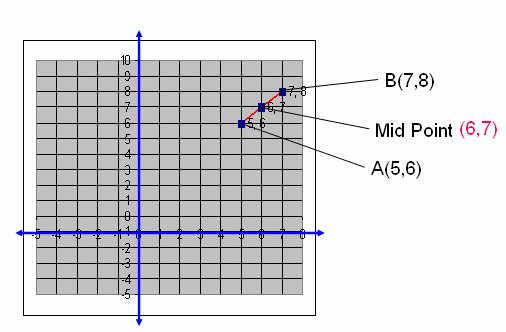Email us to get an instant 20% discount on highly effective K-12 Math & English kwizNET Programs!

Online Quiz (WorksheetABCD)

Questions Per Quiz = 2 4 6 8 10

High School Mathematics - 29.8 Mid Point of the Segment Joining Two Points

 If A(x1 , y1) and B(x2 , y2) are two points, then the mid-point of the segment joining AB is [(x1 + x2)/2 , (y1 + y2)/2] i.e., (Sum of x-coordinates/2 , Sum of y-coordinates/2). Example: Find the mid point of (5,6) and (7,8). Solution: Formula for mid point of the segment joining two points is [(x1 + x2)/2 , (y1 + y2)/2] = [(5 + 7)/2 , (6 + 8)/2] = (12/2 , 14/2) = (6,7) Therefore, the mid point of (5,6) and (7,8) is (6,7). Showing the above problem in the Graph as below.Directions: Find the mid point of the segment joining two points and draw a graph each of the following questions. Also write at least 5 examples of your own.
 Q 1: Find the mid point of the segment joining the points (4,8) and (2,4).(4,2)(3,6)(2,4)(6,3) Q 2: Find the mid point of the segment joining the points (7,8) and (11,12).(9,8)(8,9)(10,9)(9,10) Question 3: This question is available to subscribers only! Question 4: This question is available to subscribers only!